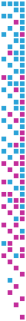# Analysis of the Quasi-Monte Carlo Integration of the Rendering Equation

László Szirmay-Kalos, Werner Purgathofer
Analysis of the Quasi-Monte Carlo Integration of the Rendering Equation
TR-186-2-98-24, August 1998

## Information

• Publication Type: Technical Report
• Workgroup(s)/Project(s): not specified
• Date: August 1998
• Number: TR-186-2-98-24
• Keywords: Hardy-Krause vari ation, quasi-Monte Carlo quadrature, Rendering equation

## Abstract

Quasi-Monte Carlo integration is said to be better than Monte-Carlo integration since its error bound can be in the order of $O(N^{-(1-\epsilon)})$ instead of the $O(N^{-0.5})$ probabilistic bound of classical Monte-Carlo integration if the integrand has finite variation. However, since in computer graphics the integrand of the rendering equation is usually discontinous and thus has infinite variation, the superiority of quasi-Monte Carlo integration has not been theoretically justified. This paper examines the integration of discontinuous functions using both theoretical arguments and simulations and explains what kind of improvements can be expected from the quasi-Monte Carlo techniques in computer graphics.

No further information available.

## BibTeX

@techreport{Szir-1998-QMC,
title =      " Analysis of the Quasi-Monte Carlo Integration of the
Rendering Equation",
author =     "L\'{a}szl\'{o} Szirmay-Kalos and Werner Purgathofer",
year =       "1998",
abstract =   "Quasi-Monte Carlo integration is said to be better than
Monte-Carlo integration since its error bound can be in the
order of $O(N^{-(1-\epsilon)})$ instead of the $O(N^{-0.5})$
probabilistic bound of classical Monte-Carlo integration if
the integrand has finite variation. However, since in
computer graphics the integrand of the rendering equation is
usually discontinous and thus has infinite variation, the
superiority of quasi-Monte Carlo integration has not been
theoretically justified. This paper examines the integration
of discontinuous functions using both theoretical arguments
and simulations and explains what kind of improvements can
be expected from the quasi-Monte Carlo techniques in
computer graphics.",
month =      aug,
number =     "TR-186-2-98-24",
address =    "Favoritenstrasse 9-11/E193-02, A-1040 Vienna, Austria",
institution = "Institute of Computer Graphics and Algorithms, Vienna
University of Technology ",
note =       "human contact: technical-report@cg.tuwien.ac.at",
keywords =   "Hardy-Krause vari ation, quasi-Monte Carlo quadrature,
Rendering equation",
URL =        "https://www.cg.tuwien.ac.at/research/publications/1998/Szir-1998-QMC/",
}